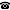# 2014 seminar talk: One Hierarchy Spawns Another: The Complexity Classification of Conjunctive Queries

Talk held by Hubie Chen (Universidad del País Vasco, San Sebastian, Spain) at the KGRC seminar on 2014-03-20.

### Abstract

We study the problem of conjunctive query evaluation over sets of queries; this problem is formulated here as the relational homomorphism problem over a class of structures $A$, wherein each instance must be a pair of structures such that the first structure is an element of $A$.

We introduce a notion which we call graph deconstruction, which is a variant of the well-known notion of tree decomposition.  Using this notion, we give a novel, modular, and conceptually clean presentation of the theorem describing the tractable homomorphism problems in the framework under study (assuming bounded arity).

We then study a binary relation on graph classes that is naturally induced by the notion of graph deconstruction.  We completely describe the hierarchy of graph classes that this binary relation yields, and discuss how this hierarchy of graph classes can be used to infer a complexity-theoretic hierarchy of homomorphism problems, which is extremely fine as it is exhaustive up to a computationally very weak notion of reduction (namely, a parameterized form of quantifier-free reduction).

We also plan to present and develop a theory of Ehrenfeucht-Fraisse-style pebble games for solving cases of the homomorphism problem wherein the tree depth is bounded.

A version of the article on which this talk is based is available at: http://arxiv.org/abs/1307.1353

Kurt Gödel Research Center for Mathematical Logic. Währinger Straße 25, 1090 Wien, Austria.+43-1-4277-50501. Last updated: 2010-12-16, 04:37.